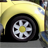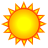# get vertex coordinates

8462
12
02-08-2012 02:43 AMby
New Contributor III
Hi,

it's been a while since my last python scripting - now I need to solve this problem and I'm stuck 😞 I know it is something easy, but I can't make it work, so I'm asking for help... I want to get coordinates (X and Y) of each vertex of line - that is easy with http://help.arcgis.com/en/arcgisdesktop/10.0/help/index.html#/Reading_geometries/002z0000001t000000/ - but in every loop I need to get coordinates of next two point as well (for making some calculations) and that is my problem....

Any help with this little problem please?

Thank you so much!

Bart
Tags (3)
1 Solution

Accepted SolutionsRegular Contributor III
Sure you can do it in the cursor... I guess an update cursor so you could then populate your angle statistic field value, right?

It'd look something like this then:

`#assuming all features are single part!!! import arcpy lineFC = r"C:\temp\test.shp" dsc = arcpy.Describe(lineFC) shapeFieldName = dsc.ShapeFieldName featureNumber = 0 updateRows = arcpy.UpdateCursor(lineFC) for updateRow in updateRows:    vertexList = []    shapeObj = updateRow.getValue(shapeFieldName)    partObj = shapeObj.getPart(0)    for pointObj in partObj:       vertexList.append((pointObj.X, pointObj.Y))    vertexCount = len(vertexList)    if vertexCount < 3:       break #if only two verticies in the line, break the loop since there is no "angle" to calculate    i = 0 #    while i + 3 <= vertexCount: #that is until you run out of vertex triplicate pairs to count        x1 = vertexList       y1 = vertexList       x2 = vertexList[i + 1]       y2 = vertexList[i + 1]       x3 = vertexList[i + 2]       y3 = vertexList[i + 2]       funkyStat = (x1 + y2) / 1.61803399 #blah blah whatever formula       i = i + 1    updateRow.FUNKY_STAT = funkyStat    updateRows.updateRow(updateRow) del updateRow, updateRows`
12 RepliesRegular Contributor III
You could read the verticies into a python list... actually a series of imbedded lists. For example:

```#assuming all features are single part!!!
import arcpy
fcVertexList = []
lineFC = r"C:\temp\test.shp"
dsc = arcpy.Describe(lineFC)
shapeFieldName = dsc.ShapeFieldName
featureNumber = 0
searchRows = arcpy.SearchCursor(lineFC)
for searchRow in searchRows:
featureNumber = featureNumber + 1
fcVertexList.append([])
shapeObj = searchRow.getValue(shapeFieldName)
partObj = shapeObj.getPart(0)
for pointObj in partObj:
fcVertexList[featureNumber - 1].append([pointObj.X, pointObj.Y])```

Then it's just a simple matter of slicing a Python list:

To retreive all the verticies for the 1st feature:
>>> print fcVertexList

To cound how many verticies are in the 1st feature:
>>> print len(fcVertexList)

To retreive the first three verticies for the first feature:
>>> fcVertexList[0:3]

To retreive the next three verticies (incremented by 1 vertex) for the first feature:
>>> fcVertexList[1:4]by
New Contributor III
Thank you Chris for your answer. I know I can store coordinates of verticies in a list and then just simply read what I want, but I was wondering if there is any "smarter" way... if it is quite a big shapefile/feature class (in my case - roads), it takes some time to write all coordinates into list and it takes quite a lot of memory. What I need is to calculate some characteristics of each line (analytical geometry - vectors and so... in my case I want to get the "angle change" of each line) and store it in new field - it should be better to calculate the characteristic directly, without writing coordinates into list, like "take line - get coordinates of points 1-3 - calculate angle change - store the value - move to points 2-3 and repeat..."... Is this possible? (Or, just to be sure - is there any better way to calculate the "angle change" of each line?:))Occasional Contributor III
You could use a list that only contains the 3 vertices in question. As you traverse each line, after calculating the values for each vertex, use list.pop(0) to remove the oldest vertex and list.append() to add the new. That should simplify your equations, because they will only reference list, list, and list.Regular Contributor III
Sure you can do it in the cursor... I guess an update cursor so you could then populate your angle statistic field value, right?

It'd look something like this then:

`#assuming all features are single part!!! import arcpy lineFC = r"C:\temp\test.shp" dsc = arcpy.Describe(lineFC) shapeFieldName = dsc.ShapeFieldName featureNumber = 0 updateRows = arcpy.UpdateCursor(lineFC) for updateRow in updateRows:    vertexList = []    shapeObj = updateRow.getValue(shapeFieldName)    partObj = shapeObj.getPart(0)    for pointObj in partObj:       vertexList.append((pointObj.X, pointObj.Y))    vertexCount = len(vertexList)    if vertexCount < 3:       break #if only two verticies in the line, break the loop since there is no "angle" to calculate    i = 0 #    while i + 3 <= vertexCount: #that is until you run out of vertex triplicate pairs to count        x1 = vertexList       y1 = vertexList       x2 = vertexList[i + 1]       y2 = vertexList[i + 1]       x3 = vertexList[i + 2]       y3 = vertexList[i + 2]       funkyStat = (x1 + y2) / 1.61803399 #blah blah whatever formula       i = i + 1    updateRow.FUNKY_STAT = funkyStat    updateRows.updateRow(updateRow) del updateRow, updateRows`by
New Contributor III
Sure you can do it in the cursor... I guess an update cursor so you could then populate your angle statistic field value, right?

It'd look something like this then:

...

Thanks, my funky statistic 😉 is working perfectly that way... (just had a little problem with "break", but that's already solved)..

Thank to all of you for your help!Regular Contributor III

A request for you: Many of us have an interest in seeing this forum also serve as a sort of a code/algorithm library... That said, it sounds like the statistic you are calculating is some sort of "maximum angle" statistic. If so, would you mind posting your code? Even if not, it sounds interesting, and I bet a lot of people would find it useful. If not, that's okay too.by
New Contributor III
Sorry for late response - so much another work to do:( Ok, I understand, no problem with posting the code. Just to make it clear - it calculates "average curvature" of each line (angular change devided by length of line). I'm pretty sure there is a room for improvement in the script, I just did not have enough time to work on it...

```#
# This script calculates average "curvature" of roads
#
# It takes a line feature class (with pre-added fields "angleChng" and "AvCurv"!)
# then takes the fist line
# then takes first three verticies
# and calculates the angular change of line between those three verticies
# then it loops through all verticies of the line, calculates angular changes and cumulates them
# after going through whole line, it writes down the total angular change, calculates and writes down the average curvature
# and then this is repeated for all lines in the feature class
#

import arcpy, math, datetime, numpy
from arcpy import env
print "starting"
start = datetime.datetime.now() # for calculating time of process
lineFC = r"roads.shp" # input fc - with pre-added fields "angleChng" and "AvCurv"!
dsc = arcpy.Describe(lineFC)
shapeFieldName = dsc.ShapeFieldName
updateRows = arcpy.UpdateCursor(lineFC)
for updateRow in updateRows: # looping through lines
vertexList = []
shapeObj = updateRow.getValue(shapeFieldName)
partObj = shapeObj.getPart(0)
for pointObj in partObj:
vertexList.append((pointObj.X, pointObj.Y)) # getting coordinates of all line vertices
vertexCount = len(vertexList)
i = 0
angularChange=0
averageCurvature=0
while i + 3 <= vertexCount: # that is until you run out of vertex triplicate pairs to count
x1 = vertexList # coordinates of three vertices
y1 = vertexList
x2 = vertexList[i + 1]
y2 = vertexList[i + 1]
x3 = vertexList[i + 2]
y3 = vertexList[i + 2]
u1=x1-x2 # vectors (BA and BC !)
u2=y1-y2
v1=x3-x2
v2=y3-y2
cit=(u1*v1)+(u2*v2) # calculating the angle of vectors
jmen1=math.sqrt((u1*u1)+(u2*u2))
jmen2=math.sqrt((v1*v1)+(v2*v2))
jmen=jmen1*jmen2
cosfi=cit/jmen
angularChange=angularChange+fiGrad # total angle change of line
i = i + 1
averageCurvature=angularChange/shapeObj.length*1000 # average curvature of line = angle change in one kilometer
updateRow.angleChng=angularChange # adding angle change of line
updateRow.AvCurv=averageCurvature # adding average curvature of line
updateRows.updateRow(updateRow)
print "Done in ",datetime.datetime.now() - start, " seconds"
del updateRows, updateRow, lineFC```

Btw.: Maybe a newbie question, but i didn't find any solution yet - Is it possible to add field into fc (with fieldName as a variable) and the to use something like updateRow.fieldName? (if using posted script, it is necessary to have "pre-added" fields - it would be much easier to add them in the beginning of the script...) Thanks!Regular Contributor III
Very nice - This is actually quite usefull to me - I have done some projects in the past doing network anaylysis on logging roads... And I was looking for a way to apply some factors that would describe how "difficult" the road is to manuver (road type as well as each segment's vertical/horizontal complexity). When I get back to my routing project (months away), I'll try to post some code that uses z values as well. Thanks for posting the code...Regular Contributor III
Maybe a newbie question, but i didn't find any solution yet - Is it possible to add field into fc (with fieldName as a variable) and the to use something like updateRow.fieldName? (if using posted script, it is necessary to have "pre-added" fields - it would be much easier to add them in the beginning of the script...) Thanks!

If the field is a variable, you have to use the .getValue (for read access) or .setValue (for write access) methods. For example:

```field1 = "FIELD1"
field2 = "FIELD2"
updateRows = arcpy.UpdateCursor(fc)
for updateRow in updateRows:
field1Value = updateRow.getValue(field1)
updateRow.setValue(field2, field1Value / 2)
updateRows.updateRow(updateRow)
del updateRow, updateRows```